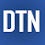## Pages

### Support Vector Regression Example in Python

Support Vector Regression (SVR) is a regression algorithm and it applies a similar technique of Support Vector Machines (SVM) for regression analysis. As we know, regression data contains continuous real numbers. To fit such type of data, the SVR model approximates the best values with a given margin called ε-tube (epsilon-tube, ε identifies a tube width) with considering the model complexity and error rate.
In this tutorial, we'll briefly learn how to fit and predict regression data with SVR method by using SVR class of Scikit-learn API in Python. The tutorial covers:
1. Preparing the data
2. Model fitting and prediction
3. Accuracy check
4. Source code listing
5. Video tutorial

```import numpy as np
from sklearn.svm import SVR
from sklearn.metrics import mean_squared_error
import matplotlib.pyplot as plt```

Preparing the data

We'll use randomly generated regression data as a target data to fit. He, we can write simple function to generate data.

```np.random.seed(21)

N = 1000
def makeData(x):
r = [a/10 for a in x]
y = np.sin(x)+np.random.uniform(-.5, .2, len(x))
return np.array(y+r)

x = [i/100 for i in range(N)]
y = makeData(x)
x = np.array(x).reshape(-1,1)

plt.scatter(x, y, s=5, color="blue")
plt.show()```

Model fitting and prediction

We'll use Scikit-learn API's SVR class to define the model. The model can be used with default parameters. We'll fit the model on x and y data.

```svr = SVR().fit(x, y)
print(svr)```

SVR(C=1.0, cache_size=200, coef0=0.0, degree=3, epsilon=0.1, gamma='auto_deprecated', kernel='rbf', max_iter=-1, shrinking=True, tol=0.001, verbose=False)

Here, kernel, C, and epsilon parameters can be changed according to regression data characteristics. Kernel identifies kernel type in an algorithm. An 'rbf' (default kernel), 'linear', 'poly', and 'sigmoid' can be used.

Next, we'll predict x data with svr model.

`yfit = svr.predict(x)`

To check the predicted result, we'll visualize the both y and yfit data in a plot.

```plt.scatter(x, y, s=5, color="blue", label="original")
plt.plot(x, yfit, lw=2, color="red", label="fitted")
plt.legend()
plt.show()```

Accuracy check

Finally, we'll check the model and prediction accuracy with metrics of R-squared and MSE.

```score = svr.score(x,y)
print("R-squared:", score)
print("MSE:", mean_squared_error(y, yfit))```

R-squared: 0.9211937698347702 MSE: 0.0411375232810873

In this tutorial, we've briefly learned how to fit regression data by using the SVR method in Python. The full source code is listed below.

Source code listing

```import numpy as np
from sklearn.svm import SVR
from sklearn.metrics import mean_squared_error
import matplotlib.pyplot as plt

np.random.seed(21)

N = 1000
def makeData(x):
r = [a/10 for a in x]
y = np.sin(x)+np.random.uniform(-.5, .2, len(x))
return np.array(y+r)

x = [i/100 for i in range(N)]
y = makeData(x)
x = np.array(x).reshape(-1,1)

plt.scatter(x, y, s=5, color="blue")
plt.show()

svr = SVR().fit(x, y)
print(svr)

yfit = svr.predict(x)

plt.scatter(x, y, s=5, color="blue", label="original")
plt.plot(x, yfit, lw=2, color="red", label="fitted")
plt.legend()
plt.show()```

```score = svr.score(x,y)
print("R-squared:", score)
print("MSE:", mean_squared_error(y, yfit))```

Video tutorial

1.its really great tutorial.

2.Thank you, well done!

3.Hi, why is the red line is called predicted, isn't this line an approximation? Can SVR actually can be used to predict? let's say I have 2 minutes of data, can I apply SVR to predict how is this data going to behave for the next 20 minutes?

1.no because train should always be larger than size of data you try to predict

4.5.good but can be better

1.can you do better

6.It an excellent tutorial. Can I use this example in my lecture? Will it have copyright issues?

1.Thank you! Yes you can use it. Please mention this blog as a source of your content.

7.what about if we have multiple regressors